Courses

Electrostatic Practice Level - 1

30 Questions MCQ Test | Electrostatic Practice Level - 1

Description
Attempt Electrostatic Practice Level - 1 | 30 questions in 60 minutes | Mock test for Class 12 preparation | Free important questions MCQ to study for Class 12 Exam | Download free PDF with solutions
QUESTION: 1

Four charges equal to - Q are placed at the four corners of a square and a charge q is at its center. If the system is in equilibrium the value of q is

Solution: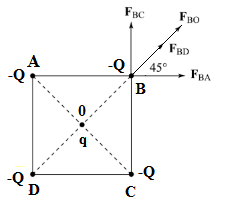If each charge experiences no force then the system is in equilibrium.
From the fihure it is clear that the charge 'q' at the cnetre would be in equilibrium for any value of q. Let AB = BC = CD = DA = a.
From the figure, we get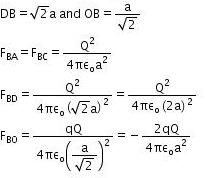The net force acting on - Q at point B will be zero if
FBACOS 45 + FBCcos 45 + FBD + FBO = O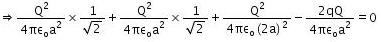On solving, we get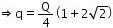QUESTION: 2

Solution:
QUESTION: 3

Under the action of a given coulombic force the acceleration of an electron is 2.5 × 1022 m/s2. Then the magnitude of the acceleration of a proton under the action of same force is nearly

Solution:
QUESTION: 4

Find the susceptibility of a material whose dielectric constant is 2.26.

Solution:

Electric susceptibility is the measure of ability of the material to get polarised. It is given by, χe = εr – 1.Thus we get 1.26.

QUESTION: 5

What is the total charge in coulomb of 75.0 kg of electrons?

Solution:
QUESTION: 6

Under the influence of the coulomb field of charge +Q, a charge - q is moving around it in an elliptical orbit. Find out the correct statements(s).

Solution:
QUESTION: 7

A charge Q is placed at each of the opposite corners of a square. A charge q is placed at each of the other two corners. If the net electrical force on Q is zero, then the Q/q equals.

Solution:
QUESTION: 8

An object A has a charge of -2μC and the object B has a charge of +6μC. Which statement is true?

Solution:
QUESTION: 9

A charge of 1 μC is divided into two parts such that their charges are in the ratio of 2 : 3. These two charges are kept at a distance 1 m apart in vacuum. Then, the electric force between them (in N) is

Solution:
QUESTION: 10

Two identical positive charges are fixed on the y-axis at equal distances from the origin O. A negatively charged particle starts on the x-axis, at a large distance from O, moves along the x-axis, passes through O and moves far away from O. Its acceleration A is taken as positive along its direction of motion. The best graph between the particle's acceleration and its x-coordinate is represented by

Solution:
QUESTION: 11

Two identical metal spheres charged with +12μF and -8μF are kept at certain distance in air. They are brought into contact and then kept at the same distance. The ratio of the magnitudes of electrostatic forces between them before and after contact is

Solution:
QUESTION: 12

The force of interaction between two charges q1 = 6μC and q2 = 2μC is 12 N. If charge q = -2μC is added to each of the charges, then the new force of interaction is

Solution:
QUESTION: 13

In nature, the electric charge of any system is always equal to

Solution:
QUESTION: 14

A comb run through one's dry hair attracts small bits of paper. This is due to

Solution:
QUESTION: 15

A and B are two identical spherical charged bodies which repel each other with force F, kept at a finite distance. A third uncharged sphere of the same size is brought in contact with sphere B and removed. It is then kept at mid-point of A and B. Find the magnitude of force on C.

Solution:
QUESTION: 16

Two equal charges as separated by a distnce d. A third charge placed on a perpendicular bisector at x distance from center will experience maximum coulomb force. when.

Solution:
QUESTION: 17

Charges are placed at corners of a square of side a as shown in the following figure. The charge A is in equilibrium. The ratio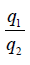is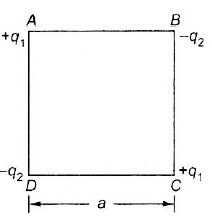Solution:
QUESTION: 18

Consider a neutral conducting sphere. A positive point charge is placed outside the sphere. The net charge on the sphere is then

Solution:
QUESTION: 19

A charge Q is divided in two parts q and Q - q. What is value of q for maximum force between them?

Solution:
QUESTION: 20

Two identical charges repel each other with a force equal to 10 mg wt when they are 0.6 m apart in air (g = 10 ms-2) The value of each charge is

Solution:
QUESTION: 21

Two unit negative charges are placed on a straight line. A positive charge q is placed exactly at the mid-point between these unit charges. If the system of these three charges is in equilibrium, the value of q (in C) is

Solution:
QUESTION: 22

Two spherical conductors B and C having equal radii and carrying equal charges in them repel each other with a force F when kept apart at some distance. A third spherical conductor having same radius as that of B but uncharged, is brought in contact with B, then brought in contact with C and finally removed away from both. The new force of repulsion between B and C is

Solution:
QUESTION: 23

Three charges 1μC, 1μC and 2μC are kept at vertices of A, B and C of an equilateral triangle ABC of 10 cm sie respectively, The resultant force of the charge at C is

Solution:
QUESTION: 24

Force between two identical charges placed at a distance of r in vacuum is F. Now a slab of dielectric of dielectric constant 4 is inserted between these two charges. If the thickness of the slab is r/2, then the force between the charges will become

Solution:
QUESTION: 25

Identify the wrong statement in the following. Coulomb's law correctly described the electric force that

Solution:
QUESTION: 26

If a conducting medium is placed between two charges, then the electric force between them will become

Solution:
QUESTION: 27

A charge q is placed at the centre of the line joining two equal point charges each equal to Q. The system of three charges will be in equilibrium if q is equal to

Solution:
QUESTION: 28

An infinite number of charges, each of charge, 1μC, are placed on the x-axis with co-ordinates x = 1, 2, 4, 8, ... ∞. If a charge of 1c is kept at the origin, then what is the net force acting on 1 C charge?

Solution:
QUESTION: 29

Two charges of equal magnitudes and at a distance r exert a force F on each other. If the cahrges are halved and distance between them is doubled, then the new force acting on each charge is

Solution:
QUESTION: 30

When a body is earth connected, electrons from the earth flow into the body. This means the body is

Solution:Use Code STAYHOME200 and get INR 200 additional OFF Use Coupon Code

Track your progress, build streaks, highlight & save important lessons and more!

Similar ContentRelated tests